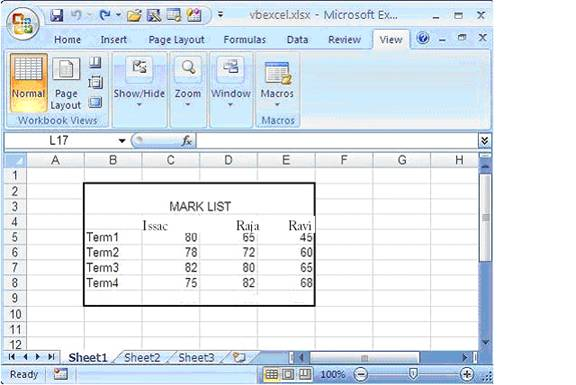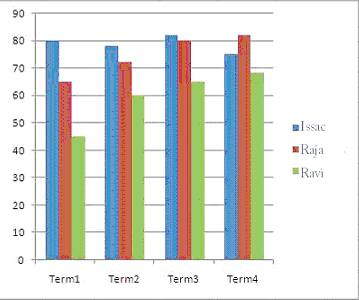# Programming Tutorials

## Export Chart from Excel 2007 as Picture file in VB.NET 2005

By: Issac

The following program shows how can Export a Chart from Excel 2007 to Picture file like , BMP , JPG , GIF etc .Before we crate a Chart , we have to fill data in Excel sheet. After enter data your Excel sheet is look like the following picture.After filling the data, We have to create a chart object in VB.NET and configure the Chart object with necressary data like positions, size, data range, chart type etc.. And use the command for export chart as picture file

The following picture shows the BMP file export from Excel.``````Imports Excel = Microsoft.Office.Interop.Excel
Public Class Form1
Private Sub Button1_Click(ByVal sender As System.Object, _
ByVal e As System.EventArgs) Handles Button1.Click

Dim xlApp As Excel.Application
Dim xlWorkBook As Excel.Workbook
Dim xlWorkSheet As Excel.Worksheet
Dim misValue As Object = System.Reflection.Missing.Value

xlApp = New Excel.ApplicationClass
xlWorkSheet = xlWorkBook.Sheets("sheet1")

xlWorkSheet.Cells(1, 1) = ""
xlWorkSheet.Cells(1, 2) = "Issac"
xlWorkSheet.Cells(1, 3) = "Raja"
xlWorkSheet.Cells(1, 4) = "Ravi"

xlWorkSheet.Cells(2, 1) = "Term1"
xlWorkSheet.Cells(2, 2) = "80"
xlWorkSheet.Cells(2, 3) = "65"
xlWorkSheet.Cells(2, 4) = "45"

xlWorkSheet.Cells(3, 1) = "Term2"
xlWorkSheet.Cells(3, 2) = "78"
xlWorkSheet.Cells(3, 3) = "72"
xlWorkSheet.Cells(3, 4) = "60"

xlWorkSheet.Cells(4, 1) = "Term3"
xlWorkSheet.Cells(4, 2) = "82"
xlWorkSheet.Cells(4, 3) = "80"
xlWorkSheet.Cells(4, 4) = "65"

xlWorkSheet.Cells(5, 1) = "Term4"
xlWorkSheet.Cells(5, 2) = "75"
xlWorkSheet.Cells(5, 3) = "82"
xlWorkSheet.Cells(5, 4) = "68"

'create chart
Dim chartPage As Excel.Chart
Dim xlCharts As Excel.ChartObjects
Dim myChart As Excel.ChartObject
Dim chartRange As Excel.Range

xlCharts = xlWorkSheet.ChartObjects
myChart = xlCharts.Add(10, 80, 300, 250)
chartPage = myChart.Chart
chartRange = xlWorkSheet.Range("A1", "d5")
chartPage.SetSourceData(Source:=chartRange)
chartPage.ChartType = Excel.XlChartType.xlColumnClustered

'exporting chart as picture file
xlWorkSheet.ChartObjects(1).chart.Export(FileName:= _
"C:\excel_chart_export.bmp", FilterName:="BMP")

xlWorkSheet.SaveAs("C:\vbexcel.xlsx")
xlWorkBook.Close()
xlApp.Quit()

releaseObject(xlApp)
releaseObject(xlWorkBook)
releaseObject(xlWorkSheet)

MsgBox("Chart File Exported !")
End Sub

Private Sub releaseObject(ByVal obj As Object)
Try
System.Runtime.InteropServices.Marshal.ReleaseComObject(obj)
obj = Nothing
Catch ex As Exception
obj = Nothing
Finally
GC.Collect()
End Try
End Sub
End Class``````

This source code exports a picture to Excel file